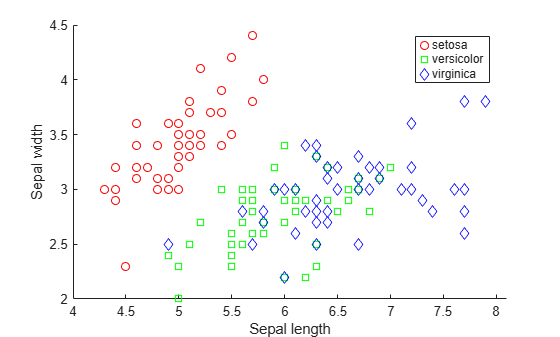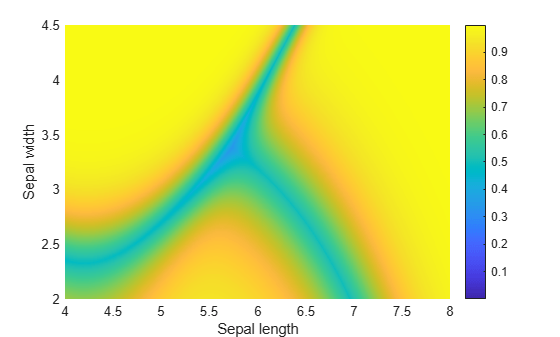# Plot Posterior Classification Probabilities

This example shows how to visualize posterior classification probabilities predicted by a naive Bayes classification model.

```load fisheriris X = meas(:,1:2); Y = species; labels = unique(Y);```

`X` is a numeric matrix that contains two petal measurements for 150 irises. `Y` is a cell array of character vectors that contains the corresponding iris species.

Visualize the data using a scatter plot. Group the variables by iris species.

```figure; gscatter(X(:,1), X(:,2), species,'rgb','osd'); xlabel('Sepal length'); ylabel('Sepal width'); ```Train a naive Bayes classifier.

`mdl = fitcnb(X,Y);`

`mdl` is a trained `ClassificationNaiveBayes` classifier.

Create a grid of points spanning the entire space within some bounds of the data. The data in `X(:,1)` ranges between 4.3 and 7.9. The data in `X(:,2)` ranges between 2 and 4.4.

```[xx1, xx2] = meshgrid(4:.01:8,2:.01:4.5); XGrid = [xx1(:) xx2(:)];```

Predict the iris species and posterior class probabilities of each observation in `XGrid` using `mdl`.

`[predictedspecies,Posterior,~] = predict(mdl,XGrid);`

Plot the posterior probability distribution for each species.

```sz = size(xx1); s = max(Posterior,[],2); figure hold on surf(xx1,xx2,reshape(Posterior(:,1),sz),'EdgeColor','none') surf(xx1,xx2,reshape(Posterior(:,2),sz),'EdgeColor','none') surf(xx1,xx2,reshape(Posterior(:,3),sz),'EdgeColor','none') xlabel('Sepal length'); ylabel('Sepal width'); colorbar view(2) hold off```The closer an observation gets to the decision surface, the less probable it is that the data belongs to a certain species.

Plot the classification probability distributions individually.

```figure('Units','Normalized','Position',[0.25,0.55,0.4,0.35]); hold on surf(xx1,xx2,reshape(Posterior(:,1),sz),'FaceColor','red','EdgeColor','none') surf(xx1,xx2,reshape(Posterior(:,2),sz),'FaceColor','blue','EdgeColor','none') surf(xx1,xx2,reshape(Posterior(:,3),sz),'FaceColor','green','EdgeColor','none') xlabel('Sepal length'); ylabel('Sepal width'); zlabel('Probability'); legend(labels) title('Classification Probability') alpha(0.2) view(3) hold off```